This tutorial illustrates methods to help with masking data.

First, let’s load the pain data for this example.

```from nltools.datasets import fetch_pain

data = fetch_pain()
```

Spherical masks can be created using the create_sphere function. It requires specifying a center voxel and the radius of the sphere.

```from nltools.mask import create_sphere

```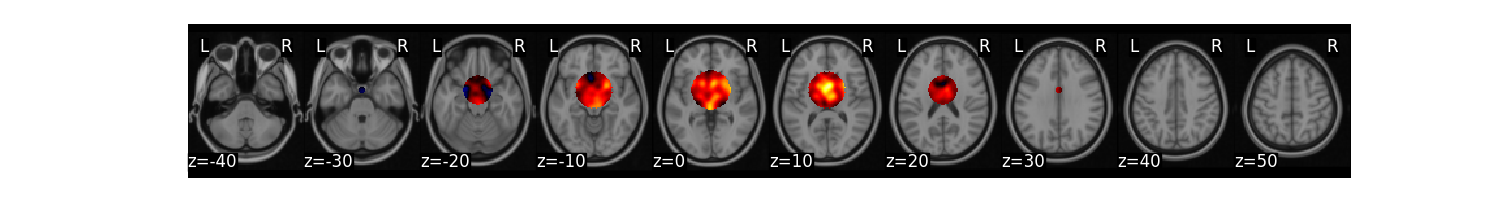Out:

```/opt/hostedtoolcache/Python/3.8.9/x64/lib/python3.8/site-packages/nilearn/image/resampling.py:273: UserWarning: Resampling binary images with continuous or linear interpolation. This might lead to unexpected results. You might consider using nearest interpolation instead.
warnings.warn("Resampling binary images with continuous or "
```

## Extract Mean Within ROI¶

We can easily calculate the mean within an ROI for each image within a Brain_Data() instance using the extract_roi() method.

```import matplotlib.pyplot as plt

plt.plot(mean)
```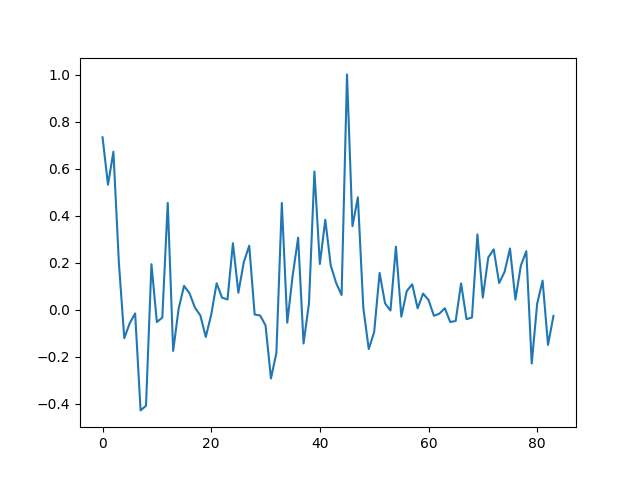Out:

```[<matplotlib.lines.Line2D object at 0x7f7cfe34bd30>]
```

## Expand and Contract ROIs¶

Some masks have many ROIs indicated by a unique ID. It is possible to expand these masks into separate ROIs and also collapse them into a single image again. Here we will demonstrate on a k=50 parcellation hosted on http://neurovault.org.

```from nltools.mask import expand_mask, collapse_mask
from nltools.data import Brain_Data

```We can expand this mask into 50 separate regions

```mask_x = expand_mask(mask)
```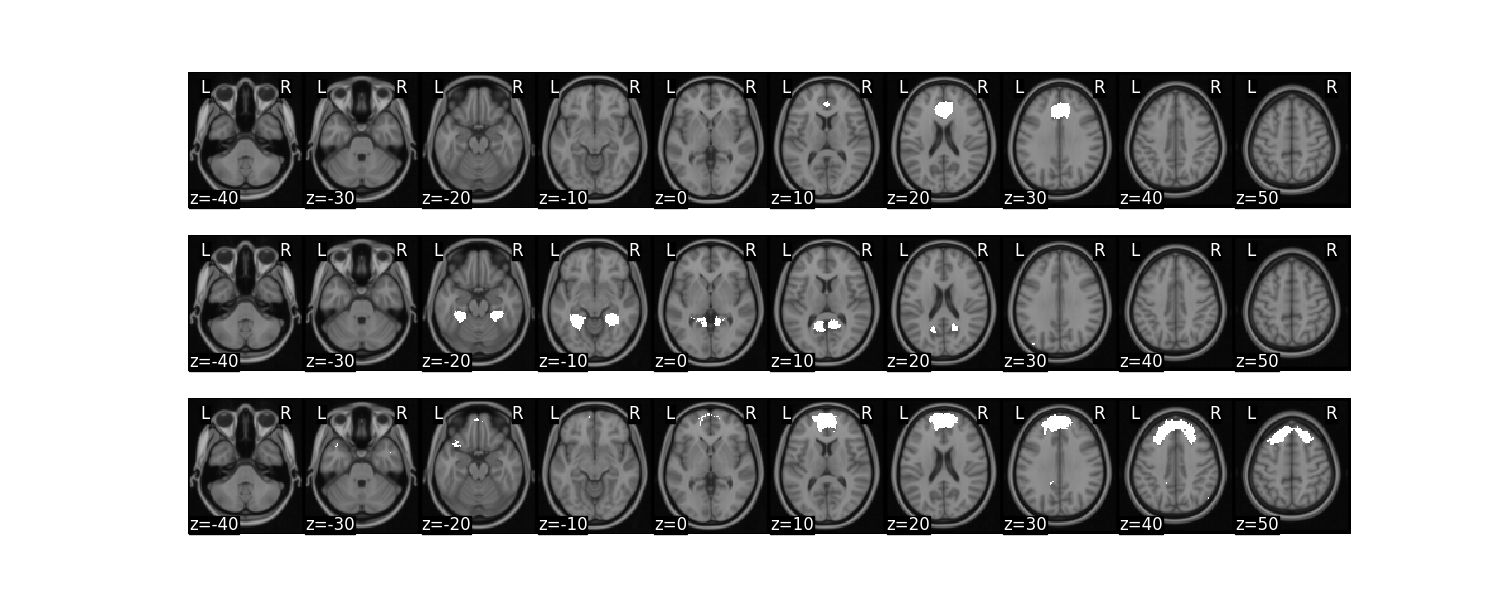We can collapse these 50 separate regions as unique values in a single image

```mask_c = collapse_mask(mask_x)
```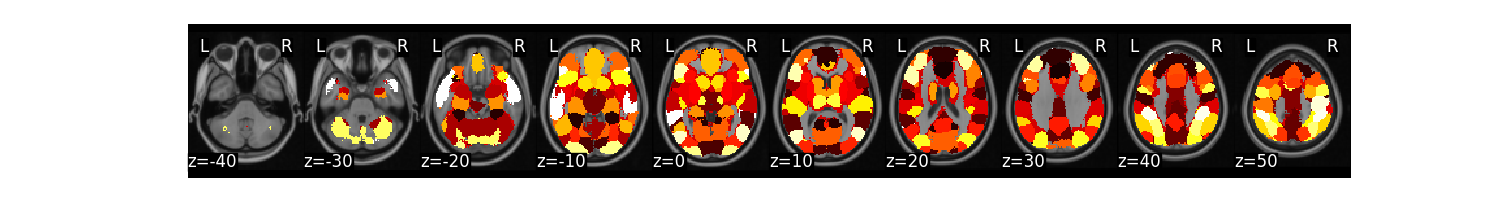## Threshold and Regions¶

Images can be thresholded using an arbitrary cutoff or a percentile using the threshold method. Here we calculate the mean of the high pain images and threshold using the 95 percentile.

```high = data[data.X['PainLevel']==3]
high.mean().threshold(lower='2.5%', upper='97.5%').plot()
```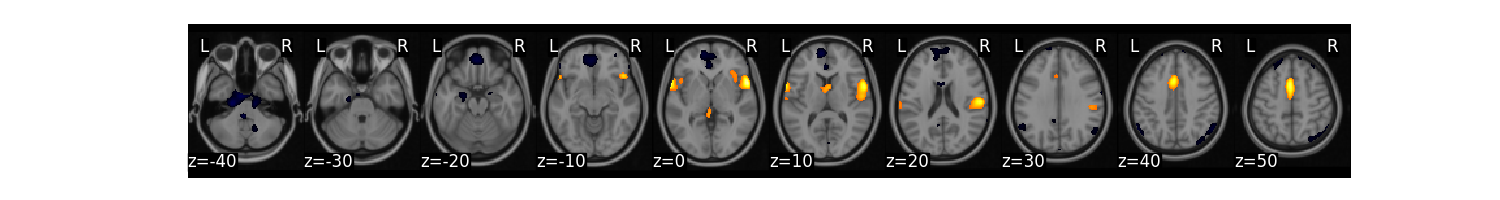We might be interested in creating a binary mask from this threshold.

```mask_b = high.mean().threshold(lower='2.5%', upper='97.5%',binarize=True)
```We might also want to create separate images from each contiguous ROI.

```region = high.mean().threshold(lower='2.5%', upper='97.5%').regions()
region.plot()
```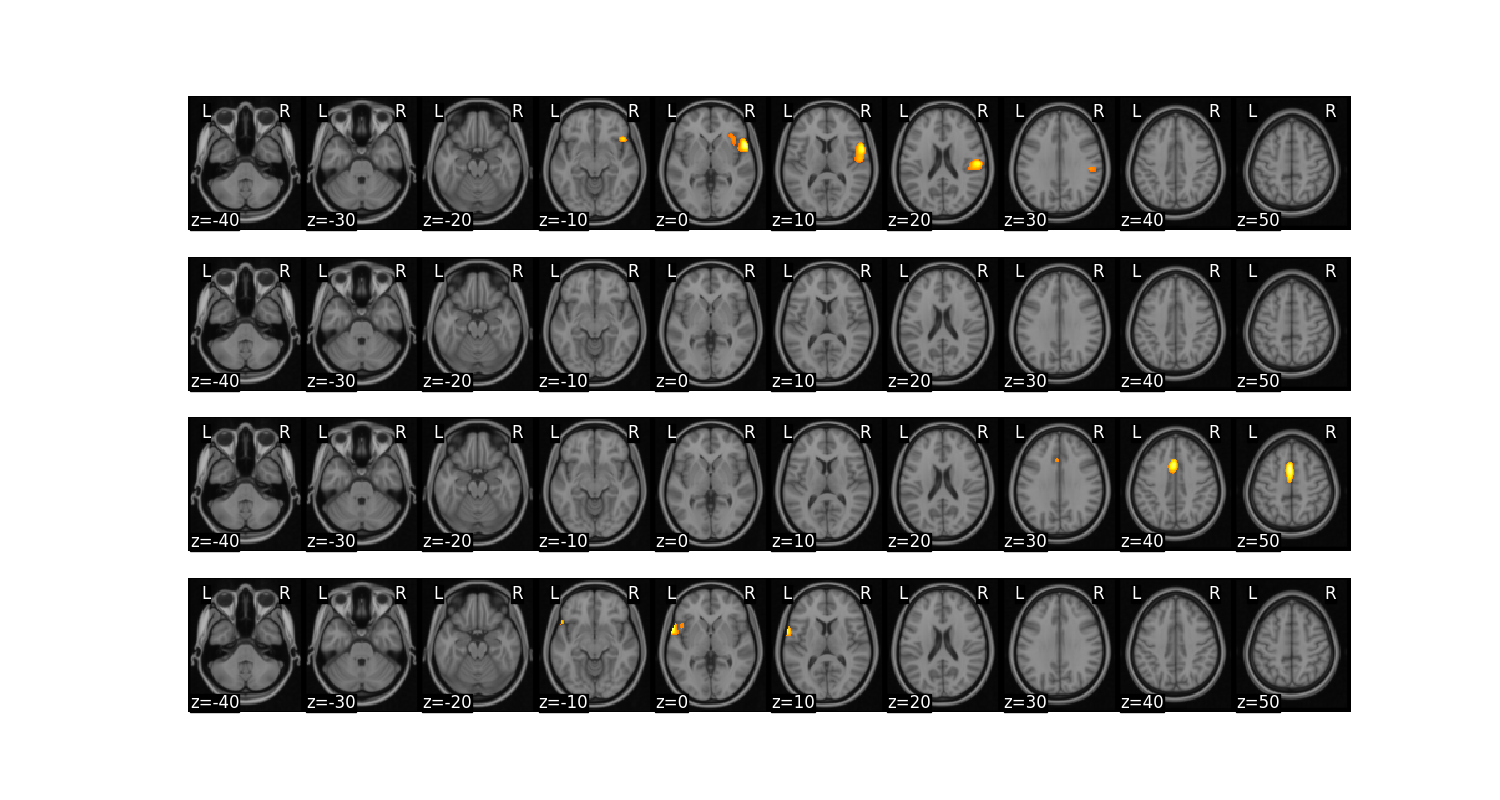Finally, we can perform operations on ROIs from a mask and then convert them back into a Brain_Data instance. In this example, let’s compute a linear contrast of increasing pain for each each participant. Then, let’s compute functional connectivity across participants within each ROI and calculate the degree centrality of each ROI after arbitrarily thresholding the connectivity matrix. We can then convert each ROIs degree back into a Brain_Data instance to help visualize which regions are more central in this analysis.

```from sklearn.metrics import pairwise_distances
import pandas as pd
import numpy as np

sub_list = data.X['SubjectID'].unique()

# perform matrix multiplication to compute linear contrast for each subject
lin_contrast = []
for sub in sub_list:
lin_contrast.append(data[data.X['SubjectID'] == sub] * np.array([1, -1,  0]))

# concatenate list of Brain_Data instances into a single instance
lin_contrast = Brain_Data(lin_contrast)

# Compute correlation distance between each ROI

# Threshold functional connectivity and convert to Adjacency Matrix. Plot as heatmap
dist.threshold(upper=.4, binarize=True).plot()

# Convert Adjacency matrix to networkX instance
g = dist.threshold(upper=.4, binarize=True).to_graph()

# Compute degree centrality and convert back into Brain_Data instance.
••